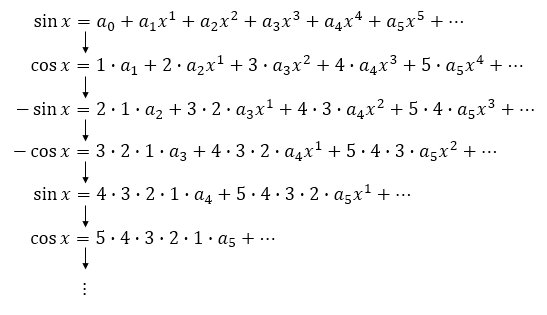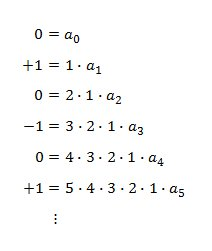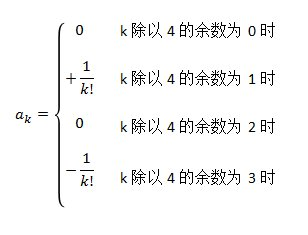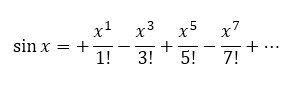• ## 康托展开和逆康托展开

万次阅读 多人点赞 2017-06-10 17:56:16
康托展开是一个全排列到一个自然数的双射，常用于构建hash表时的空间压缩。设有n个数（1，2，3，4,…,n），可以有组成不同(n!种)的排列组合，康托展开表示的就是是当前排列组合在n个不同元素的全排列中的名次。 ...


文章目录
简述原理康托展开逆康托展开示例：
应用

简述

康托展开是一个全排列到一个自然数的双射，常用于构建hash表时的空间压缩。设有n个数（1，2，3，4,…,n），可以有组成不同(n!种)的排列组合，康托展开表示的就是在n个不同元素的全排列中, 比当前排列组合小的个数，那么也可以表示当前排列组合在n个不同元素的全排列中的名次（当前的名次 = 比当前排列组合小的个数 + 1）。

原理
X=a[n]*(n-1)!+a[n-1]*(n-2)!+...+a[i]*(i-1)!+...+a*0! 其中, a[i]为整数，并且0 <= a[i] <= i, 0 <= i < n, 表示当前未出现的的元素中排第几个，这就是康托展开。
例如有3个数（1，2，3），则其排列组合及其相应的康托展开值如下：
排列组合名次康托展开值12310 * 2! + 0 * 1! + 0 * 0!013220 * 2! + 1 * 1! + 0 * 0!121331 * 2! + 0 * 1! + 0 * 0!223141 * 2! + 1 * 1! + 0 * 0!331252 * 2! + 0 * 1! + 0 * 0!432162 * 2! + 1 * 1! + 0 * 0!5
比如其中的 231：
想要计算排在它前面的排列组合数目（123，132，213），则可以转化为计算比首位小即小于2的所有排列「1 * 2！」，首位相等为2并且第二位小于3的所有排列「1 * 1！」，前两位相等为23并且第三位小于1的所有排列（0 * 0！）的和即可，康托展开为：1 * 2！+1 * 1+0 * 0=3。所以小于231的组合有3个，所以231的名次是4。
康托展开
再举个例子说明。 在（1，2，3，4，5）5个数的排列组合中，计算 34152的康托展开值。
首位是3，则小于3的数有两个，为1和2，a=2，则首位小于3的所有排列组合为 a*(5-1)!第二位是4，则小于4的数有两个，为1和2，注意这里3并不能算，因为3已经在第一位，所以其实计算的是在第二位之后小于4的个数。因此a=2第三位是1，则在其之后小于1的数有0个，所以a=0第四位是5，则在其之后小于5的数有1个，为2，所以a=1最后一位就不用计算啦，因为在它之后已经没有数了，所以a固定为0根据公式： X = 2 * 4! + 2 * 3! + 0 * 2! + 1 * 1! + 0 * 0! = 2 * 24 + 2 * 6 + 1 = 61 所以比 34152 小的组合有61个，即34152是排第62。
具体代码实现如下：（假设排列数小于10个）
static const int FAC[] = {1, 1, 2, 6, 24, 120, 720, 5040, 40320, 362880};	// 阶乘
int cantor(int *a, int n)
{
int x = 0;
for (int i = 0; i < n; ++i) {
int smaller = 0;  // 在当前位之后小于其的个数
for (int j = i + 1; j < n; ++j) {
if (a[j] < a[i])
smaller++;
}
x += FAC[n - i - 1] * smaller; // 康托展开累加
}
return x;  // 康托展开值
}


tips: 这里主要为了讲解康托展开的思路，实现的算法复杂度为O(n^2)，实际当n很大时，内层循环计算在当前位之后小于当前位的个数可以用 线段树来处理计算，而不用每次都遍历，这样复杂度可以降为O(nlogn)。

逆康托展开
一开始已经提过了，康托展开是一个全排列到一个自然数的双射，因此是可逆的。即对于上述例子，在（1，2，3，4，5）给出61可以算出起排列组合为 34152。由上述的计算过程可以容易的逆推回来，具体过程如下：
用 61 / 4! = 2余13，说明a=2,说明比首位小的数有2个，所以首位为3。用 13 / 3! = 2余1，说明a=2，说明在第二位之后小于第二位的数有2个，所以第二位为4。用 1 / 2! = 0余1，说明a=0，说明在第三位之后没有小于第三位的数，所以第三位为1。用 1 / 1! = 1余0，说明a=1，说明在第二位之后小于第四位的数有1个，所以第四位为5。最后一位自然就是剩下的数2啦。通过以上分析，所求排列组合为 34152。
具体代码实现如下：（假设排列数小于10个）
static const int FAC[] = {1, 1, 2, 6, 24, 120, 720, 5040, 40320, 362880};	// 阶乘

//康托展开逆运算
void decantor(int x, int n)
{
vector<int> rest;  // 存放当前可选数，保证有序
for(int i=1;i<=n;i++)
v.push_back(i);

vector<int> ans;  // 所求排列组合
for(int i=n;i>=1;i--)
{
int r = x % FAC[i-1];
int t = x / FAC[i-1];
x = r;
a.push_back(v[t]);      // 剩余数里第t+1个数为当前位
v.erase(v.begin()+t);   // 移除选做当前位的数
}
}

示例：
leetcode题目 60. 第k个排列直接套逆康托展开即可，需要处理的是求的是第k个排列，那么其对应的康托展开值应该减1 （k - 1）。
class Solution {
public:
// 逆康托展开
string getPermutation(int n, int k) {
static constexpr int FAC[] = {1, 1, 2, 6, 24, 120, 720, 5040, 40320, 362880};	// 阶乘

vector<int> rest;  // 存放当前可选数，有序
for(int i = 1;i <= n;i++)
rest.push_back(i);

k--;    //要先 -1 才是其康托展开的值
string ans = "";
ans.reserve(n);

for(int i = n; i >= 1; i--)
{
int r = k % FAC[i-1];
int t = k / FAC[i-1];
k = r;
ans += to_string(rest[t]);          // 剩余数里第t+1个数为当前位
rest.erase(rest.begin() + t);       // 移除选做当前位的数
}

return ans;
}
};

应用
应用最多的场景也是上述讲的它的特性。
给定一个自然数集合组合一个全排列，所其中的一个排列组合在全排列中从小到大排第几位。 在上述例子中，在（1，2，3，4，5）的全排列中，34152的排列组合排在第62位。  反过来，就是逆康托展开，求在一个全排列中，从小到大的第n个全排列是多少。 比如求在（1，2，3，4，5）的全排列中，第62个排列组合是34152。[注意具体计算中，要先 -1 才是其康托展开的值。]  另外康托展开也是一个数组到一个数的映射，因此也是可用于hash，用于空间压缩。比如在保存一个序列，我们可能需要开一个数组，如果能够把它映射成一个自然数， 则只需要保存一个整数，大大压缩空间。比如八数码问题。 
展开全文算法 acm
• 有一篇论文介绍了几种展开的方法： 《基于3D全景视觉的智能三维立体摄像设备的设计》 原始全景图像，我从另一篇博客中下载下来的： 展示一下结果： 柱面展开图： 标题 透视展开结果： 一 圆柱...


有一篇论文介绍了几种展开的方法：
《基于3D全景视觉的智能三维立体摄像设备的设计》

原始全景图像，我从另一篇博客中下载下来的：展示一下结果：
柱面展开图：

标题

透视展开结果：一  圆柱展开
cylinderOn
void  cylinderOn(Mat Src)
{
int nbottom = 0;
int ntop = 0;
int nright = 0;
int nleft = 0;

//根据边界值来获得直径
nright = Src.cols;
nleft = 0;
nbottom = Src.rows;
ntop =0;
int d = min(nright - nleft, nbottom - ntop);

Mat imgRoi;
imgRoi = Src(Rect(nleft, ntop, d, d));
imshow("ROI", imgRoi);
imwrite("ROI.jpg", imgRoi);

Mat dst(imgRoi.size(), CV_8UC3, Scalar(255, 255, 255));

//建立映射表
Mat map_x, map_y;
map_x.create(imgRoi.size(), CV_32FC1);
map_y.create(imgRoi.size(), CV_32FC1);
for (int j = 0; j < d - 1; j++)
{
for (int i = 0; i < d - 1; i++)
{
map_x.at<float>(i, j) = static_cast<float>(d / 2.0 + i / 2.0*cos(1.0*j / d * 2 * CV_PI));//计算映射后的坐标
map_y.at<float>(i, j) = static_cast<float>(d / 2.0 + i / 2.0*sin(1.0*j / d * 2 * CV_PI));
}
}
//opencv自带的重映射函数
remap(imgRoi, dst, map_x, map_y, CV_INTER_LINEAR, BORDER_CONSTANT, Scalar(0, 0, 0));//用线性插值
//重设大小
resize(dst, dst, Size(), 2.0, 1.0);

rotateImage(dst);//将图像旋转180度 映射之后是倒着的

imshow("柱面投影结果", dst);
imwrite("result.jpg", dst);
}
映射完之后旋转180度  rotateImage
void rotateImage(Mat &dst)//旋转图像
{
Point center(dst.cols / 2, dst.rows / 2);
double angle = 180;//旋转180度
double scale = 1.0;//不缩放
Mat rotMat = getRotationMatrix2D(center, angle, scale);//计算旋转矩阵
warpAffine(dst, dst, rotMat, dst.size());//生成图像
}
二 透视展开
double GetAngle(int i_ExpandWidth, int i_ExpandHeight,int outR)//获取角度
{
double dw_Angle = (double)i_ExpandWidth / (double)outR;
return dw_Angle;
}

{
return i_ExpandHeight;
}

CvPoint FindPoint(double dw_Angle, int i_Radius, int innerR, int x_dot, int y_dot, IplImage* src)
{
double x, y;
x = i_Radius * cos(dw_Angle) + x_dot;//计算新的坐标
y = i_Radius * sin(dw_Angle) + y_dot;

if (x < 0)x = 0;//判断是否超过边界 超过左边设置为0  超过右边 设置为右边坐标
if (x >= src->width)x = src->width - 1;
if (y < 0)y = 0;
if (y >= src->height)y = src->width - 1;

CvPoint pt = { (int)x,(int)y };//将点转化为整数坐标

return pt;
}

void perspectOn(IplImage* src)
{

int x_dot = 314;
int y_dot = 295;
int innerR = 50;
int outR = 310;

int Width = int(2 * PI * outR);   //展开图像的宽
int Height = outR - innerR; //展开图像的高

cout << "展开图像宽:" << Width << " 高：" << Height << endl;
int i, j;
double dw_Angle;
CvPoint pt;
IplImage*dst;

dst = cvCreateImage(cvSize(Width, Height), 8, 3);
dst->origin = 0;
cvZero(dst);

uchar *dstData = (uchar*)dst->imageData;
int step = dst->widthStep / sizeof(uchar);
uchar *data1 = (uchar*)src->imageData;
int step1 = src->widthStep / sizeof(uchar);
int channels = src->nChannels;

for (i = 0; i < Width-1; i++)
{
for (j = 0; j < Height-1; j++)
{
dw_Angle = GetAngle(i, j, outR);
pt = FindPoint(dw_Angle, i_Radius, innerR, x_dot, y_dot,src);//找转换后的坐标

dstData[j*step + i * 3 + 0] = data1[pt.y*step1+pt.x*3+0];//重新赋值
dstData[j*step + i * 3 + 1] = data1[pt.y*step1 + pt.x *3+ 1];
dstData[j*step + i * 3 + 2] = data1[pt.y*step1 + pt.x *3+ 2];
}
}

cvShowImage("透视", dst);
cvSaveImage("dst.jpg", dst);
}

main函数调用
int main()
{

IplImage* src;

cylinderOn(Src);
perspectOn(src);

waitKey();
return 0;
}

展开全文• ## Chebyshev 展开

千次阅读 2013-01-13 15:31:19
Chebyshev展开是将有限区间上的光滑函数以Chebyshev多项式为基做展开。和Taylor展开不同的是，它对展开函数的光滑性要求较低，只需连续即可。著名的Chebfun系统基础之一就是Chebyshev展开。下面是Mathematica上的一...
Chebyshev展开是将有限区间上的光滑函数以Chebyshev多项式为基做展开。和Taylor展开不同的是，它对展开函数的光滑性要求较低，只需连续即可。著名的Chebfun系统基础之一就是Chebyshev展开。下面是Mathematica上的一个简单的Chebyshev展开，展开系数使用Gauss-Chebyshev积分计算，积分的代数精度是2*M+1，这里M是展开的阶数。
(************************************************************************)
(*                         Chebyshev approximation                      *)
(************************************************************************)
ChebyshevApproximation[f_, x_, a_, b_, M_] := Module[{tmp, i, j, z, F, A},
(*M:{1,x,x^2,...,x^M}*)
(* Comment: A[j] is accurate for polynomials of degree less than 2M+1 *)
If[FreeQ[f,x],Return[f]];
tmp = f /. x -> a + (b - a)*(z + 1)/2;
If[Length[nodes] != M + 1,
(* global nodes and T to avoid reduplicate computation*)
nodes = Table[Cos[(2*i + 1)*Pi/(2*(M + 1))] // GetDigit, {i, 0, M}];
T = Table[ChebyshevT[i, nodes[[j]]], {i, 0, M}, {j, 1, M + 1}]
];
F = tmp /. z -> nodes // Expand;
For[j = 0, j <= M, j++,
A[j] = 2/(M + 1)*F.T[[j + 1]] // GetDigit;
];
tmp = A/2 + Sum[A[j]*ChebyshevT[j, z], {j, 1, M}];
tmp = tmp /. z -> -1 + 2*(x - a)/(b - a) (*// Expand//GetDigit*)
];
这里GetDigit是自定义的一个函数，其作用是保持数字的有效位，默认是100位。
(************************************************************************)
(*                             Define  GetDigit[]                       *)
(************************************************************************)
(* 2012.01.05, 12:21, happy!! *)
Naccu=100;
GetDigit[p_Plus] := Map[GetDigit, p];
GetDigit[p_List] := Map[GetDigit, p];
GetDigit[p_Complex]:=GetDigit[Re[p]]+I*GetDigit[Im[p]];
GetDigit[c_Real]    := 0 /;Abs[c] < 10^(-Naccu+1);
GetDigit[c_*f_] := GetDigit[c]*f /; NumericQ[c];
GetDigit[f_] := f /; !NumericQ[f];
GetDigit[c_] := SetPrecision[Apply[Rationalize[#1]*10^#2&,MantissaExponent[c]],Naccu]/;NumericQ[c]
Maple中的Chebyshev 级数展开，展开长度M由eps自适应决定，帮助文件说“The series computed is the ‘infinite’ Chebyshev series, truncated by dropping all terms with coefficients smaller than eps muliplied the largest coefficient”，函数chebyshev的源代码如下：
> with(numapprox);
>interface(verboseproc = 2);
>print(chebyshev);

proc(f::{algebraic,procedure},eqn::{name,name=algebraic..algebraic,algebraic..algebraic},eps::numeric,size::integer)
option Copyright (c) 1992 by the University of Waterloo. All rights reserved.;
local f_is_operator,x,r,epsilon,oldDigits,a,b,evalhfOK,fproc,err,nofun,c,intf,tol,maxcoef,k,K,s,y,flags,dim,g;
if nargs<2 or 4<nargs then
error "wrong number (or type) of arguments"
elif type(f,'series') then
error "expecting an algebraic expression not of type series"
end if;
if type(eqn,=) then
f_is_operator:=false; x,r:=op(eqn)
elif type(eqn,'name') then
f_is_operator:=false; x:=eqn; r:=-1..1
else
f_is_operator:=true; r:=eqn
end if;
if type(f,'procedure') and type(eqn,{=,'name'}) then f_is_operator:=true end if;
if nargs<3 then epsilon:=Float(1,-Digits) elif Float(1,-Digits)<=eps then epsilon:=evalf(eps) else error "eps is less than 10^(-Digits)" end if;
oldDigits:=Digits;
Digits:=Digits+length(Digits+9)+1;
a:=evalf(op(1,r));
b:=evalf(op(2,r));
if not type([a,b],'[numeric,numeric]') then error "non-numeric end-points of interval" elif b<a then a,b:=b,a end if;
try
if f_is_operator then
fproc,evalhfOK:=evalf/int/CreateProc(f,_Operator,a,b,epsilon)
else fproc,evalhfOK:=evalf/int/CreateProc(f,x,a,b,epsilon)
end if
catch "function has a pole in the interval":
error "singularity in or near interval"
catch:
error
end try;
userinfo(1,'numapprox:-chebyshev',printf("procedure for evaluation is:\\n%a\\n",eval(fproc)));
if nargs=4 then dim:=size else dim:=487 end if;
flags:=array(1..3);
Assert(not assigned(intf));
if evalhfOK and Digits<=evalhf(Digits) then
try
c:=hfarray(1..dim); intf:=evalhf(evalf/int/ccquad(fproc,a,b,epsilon,dim,var(c),var(flags),true))
catch:
userinfo(1,'numapprox:-chebyshev',nprintf("evalhf mode unsuccessful - try using software floats"))
end try
end if;
if not assigned(intf) then try c:=array(1..dim); intf:=evalf/int/ccquad(fproc,a,b,epsilon,dim,c,flags,false) catch: error  end try end if;
if type(intf,{'infinity','undefined'}) then error "singularity in or near interval" end if;
Digits:=oldDigits;
err:=flags;
nofun:=round(flags);
if flags<>0 or max(epsilon*abs(intf),0.001*epsilon)+Float(1,-Digits)<err then
if dim<=487 then
userinfo(2,'numapprox:-chebyshev',nprintf("try again using more function evaluations"));
s:=procname(fproc,convert(a,'rational')..convert(b,'rational'),epsilon,4375);
return if(type(eqn,'range'),eval(s),s(x))
else
error "singularity in or near interval"
end if
end if;
c:=map((v,d)->evalf(v/d),[seq(c[k],k=1..nofun)],nofun - 1);
maxcoef:=max(1,op(c));
tol:=1/5*epsilon*maxcoef;
for K from nofun by -1 to 1 while abs(c[K])<tol do  end do;
for k by 2 to K while abs(c[k])<tol do  end do;
if K<k and 0<K then
if f_is_operator then
error "even coefficients are zero - try f(x)/x"
elif a+1.0<>0. or b - 1.0<>0. then
userinfo(2,'numapprox:-chebyshev',nprintf("even coefficents are zero -- transform to interval -1..1"));
a:=op(1,r);
b:=op(2,r);
g:=eval(f,x=1/2*(b - a)*y+1/2*a+1/2*b);
s:=procname(g,y=-1..1,epsilon,dim);
s:=subs(y=2*x/(b - a) - (a+b)/(b - a),s)
else
userinfo(2,'numapprox:-chebyshev',nprintf("function is odd -- try f(x)/x"));
Digits:=Digits+2;
s:=procname(f/x,x=r,1/100*epsilon);
s:=numapprox:-chebmult('T'(1,x),s);
Digits:=Digits - 2;
if type(s,+) then s:=map(proc(t,tol) if abs(lcoeff(t))<tol then 0 else t end if end proc,s,tol) elif abs(lcoeff(s))<tol then s:=0 end if
end if;
s:=evalf(s);
s:=numapprox:-chebsort(s);
return s
end if;
c:=[seq(if(abs(c[k])<tol,0.,c[k]),k=1..max(K,1))];
a:=op(1,r);
b:=op(2,r);
y:=2*x/(b - a) - (a+b)/(b - a);
s:=numapprox:-chebsort(s);
if type(eqn,'range') then unapply(s,x) else s end if
end proc


展开全文• ## sinx的泰勒展开式

万次阅读 多人点赞 2019-08-19 20:18:33
sinx的泰勒展开式求解过程 思路： sin x 可以如何 “ 展开 ”?写成式子就是: 最后以省略号结束，代表 “ 无穷 ”，需要求的就是 a0，a1，a2，…… 的值，准确地说就是通项公式。然后，我们就可以开始 “ 微分 ” ...
sinx的泰勒展开式求解过程
前些天发现了一个巨牛的人工智能学习网站，通俗易懂，风趣幽默，忍不住分享一下给大家，(ﾉ´▽｀)ﾉ♪-》点击这里->一个宝藏级人工智能教程网站。
思路： sin x 可以如何 “ 展开 ”?写成式子就是: 最后以省略号结束，代表 “ 无穷 ”，需要求的就是 a0，a1，a2，…… 的值，准确地说就是通项公式。然后，我们就可以开始 “ 微分 ” 了，就是等式两边同时、不停地微分下去。左边的三角函数的微分，其实是四个一循环的:sin x ➜ cos x ➜ - sin x ➜ - cos x，再回到 sin x……我们也会注意到，凡是把右边微分后，第一项(常数)就为 0 了，也就是可以直接忽略。这样一来，等式左边在有规律地循环着，等式右边每次都减少一项。当然，x = 0 时等式也会成立，那将 x = 0 带入，将消去所有 x 指数大于 0 的项(都是 0 啊)。这样一来，就可以顺利求出 a0，a1，a2，……啦，sin 0、cos 0、- sin 0 和 - cos x 分别是 0、+1 、0、-1(显然的规律)。上面是微分的过程，下面是对于所有系数得到的等式。最后，等式左边是四个一循环，可以从除以 4 的余数来考虑(分类);然后，等是右边可以用字母来代替，就是 k! × ak，这里 k! 代表阶乘。所以说，我们可以得到一个看上去漂亮的结果:如果将系数数列 a 代入，那么偶数项都会消掉(系数为 0)，只剩下一加一减的奇数项了。这就是泰勒展开(其实泰勒展开有好几个，这里只是 sin x 的泰勒展开):想法是不是很巧妙，哈哈？我也是看别人写的。其他各种复杂函数的展开式求解也采用相同的方法，很实用哦。
展开全文• ## 循环展开

千次阅读 2015-07-29 14:26:10
循环展开：减少循环次数来提高程序性能。如果展开次数太多，反而会造成性能急剧下降。因为展开次数太多，那么运算过程中中间变量会很多，而计算机的寄存器个数是固定的，当变量个数超了寄存 器，那么变量只能存到栈...性能 算法
• 泰勒公式，也称泰勒展开式。是用一个函数在某点的信息，描述其附近取值的公式。如果函数足够平滑，在已知函数在某一点的各阶导数值的情况下，泰勒公式可以利用这些导数值来做系数，构建一个多项式近似函数，求得在这...数学基础
• ## 常用泰勒展开

万次阅读 多人点赞 2017-09-19 16:22:07
• ## 泰勒展开

千次阅读 2018-08-13 14:16:17
推荐阅读时间：5min~8min主要内容：更好的理解，并且记忆泰勒展开式我们学习泰勒展开，本质上就是为了在某个点附近，用多项式函数取近似其他函数。可能有些童鞋就要问了，既然有一个函数了，为什么还需要用多项式...数学
• ## 康托展开

万次阅读 2015-04-30 16:59:48组合数学 acm 算法
• 此时可隐藏和展开 Item 的 ListView 便应运而生，这不是一个新的控件，只是我们灵活使用造出来的用法，下边我就来实现 ListView 点击 Item 展开隐藏项，包括列表单项展开、多项展开、复杂布局展开的实现，先
• 这篇答案讲的不错 泰勒级数的展开涉及到一...那么，泰勒级数公式在不同的展开展开什么不同呢？ 1，首先，不同的展开点，其与最近的奇点的距离d是不尽相同的，因此其收敛域是不同的。因为在收敛域之外，泰勒级...数学
• 通过expandedRowRender和rowclassname实现 ...图中rowclassname代表如果展开单元格没有内容 则 加一个动态类名为no-expend 从而实现隐藏当前行的展开图标 2. table数据（模拟数据） 3. less样式 ...table antd
• 这是一个典型的康拓展开应用，首先我们先阐述一下什么是康拓展开。 （1）康拓展开 　所谓康拓展开是指把一个整数X展开成如下形式： 　X=a[n]*(n-1)!+a[n-1]*(n-2)!+...+a[i]*(i-1)!+...+a*1!+a[1
• ## 展开阅读全文

千次阅读 2020-06-12 08:26:33
展开阅读全文 不知从何时起，到处都得，展开阅读全文 问了CSDN的客服，说是为了优化加载，去解决慢、卡的问题 我信了 虽然我还是觉得就是个跟风设计 谁能告诉这对用户友好在哪，我也学习学习 ...
• 具体使用场景可以参考下图，当时失败原因下菜品多余一个时，当前行可展开；当仅有一个菜品时，当前行不可展开。 解决思路 antd table要用到的属性 expandedRowRender：额外的展开行属性，类型是个方法或者React...antd table
• ## 部分分式展开

千次阅读 2020-01-02 21:09:26
• ## 泰勒级数为什么不可以展开？

千次阅读 多人点赞 2019-01-03 15:29:13
之前写了两篇关于复数的文章了： ...泰勒级数展开的问题（关于这个问题，之前写过“使用泰勒公式进行估算时，在不同点有啥区别？”，更初级、更详细一些，感兴趣可以看下）。 1 泰勒级数展开 1....复数 阿贝尔定理 收敛半径
• ## 二元泰勒展开

万次阅读 2020-03-13 08:38:42
最近在看变分法，里面有一条公式 ...多元泰勒展开其实就是在一元泰勒展开的基础上，直接把所有的变量泰勒展开加一遍 具体的证明过程可以参考 https://blog.csdn.net/chenqihome9/article/details/86349868 ...
• 后来在地址栏上加一个参数就不会展开了。 http://XXXXX/swagger2/index.html?docExpansion 或者在swagger2的index.html资源文件配置一下docExpansion,就可以了。 const ui = SwaggerUIBundle({ url: window.lo...
• 展开全部： treeObj.expandAll(true); 关闭全部： expendAllNode();//打开所有节点 expandAllNodeFalse();//关闭所有节点 展开二级全部： zNodes = eval(msg); zTree1 = \$("#tree").zTree(setting, zNodes); /...
• ## 算法基础：康托展开与逆康托展开

千次阅读 多人点赞 2019-01-18 17:41:06
康托展开与逆康托展开 序言: 本文记录康托展开与逆康拓展开的原理以及其应用。 1.概述 举例而言，对于 1 ~ 4 的一个全排列 [1, 2, 3, 4] 和 [4, 3, 2, 1]，我们知道，从字典序而言，前者是该全排列集的第一个，...
• 直三棱柱是三棱柱的其中一种，它是指是各个侧面的高相等，底面是三角形，上表面和下...那么直三棱柱的表面展开什么形状的呢？为了弄清楚，我们可以制作直三棱柱表面展开图，下面就一起来学习用几何绘图软件制作技巧。几何画板
• 康托展开 　康托展开的公式是 X=an*(n-1)!+an-1*(n-2)!+...+ai*(i-1)!+...+a2*1!+a1*0! 其中，ai为当前未出现的元素中是排在第几个（从0开始）。 　这个公式可能看着让人头大，最好举个例子来说明一下。例如，有...c
• ## Kotlin展开运算符

千次阅读 2018-11-28 12:25:04
Kotlin展开运算符 当你需要传递一个数组参数时，在Kotlin中需要你解包数组，以便每个数组元素在函数中能作为单独的参数来调用。我们使用*号来展开这个数组。 fun main() { val list: Array&lt;String&gt; =...Kotlin 可变参数
• 因为antd可展开表格可能存在数据污染的问题，最好的解决办法是只显示当前展开行，网上现在还没有类似的demo，折腾了一早上终于整出来了，心酸打工人 以下配置对可展开表格和嵌套表格均适用，嵌套表格放在父表里 &...antd react 前端
• 在项目中使用到了可展开的table，再将antd中的代码迁移到自己项目中后，发现了一个bug： 官网中的例子是每行单独展开，互不干扰的， 但是我在引用代码后，发现我无论我点击哪个，所有的行都会展开，开始我以为是...react ant...